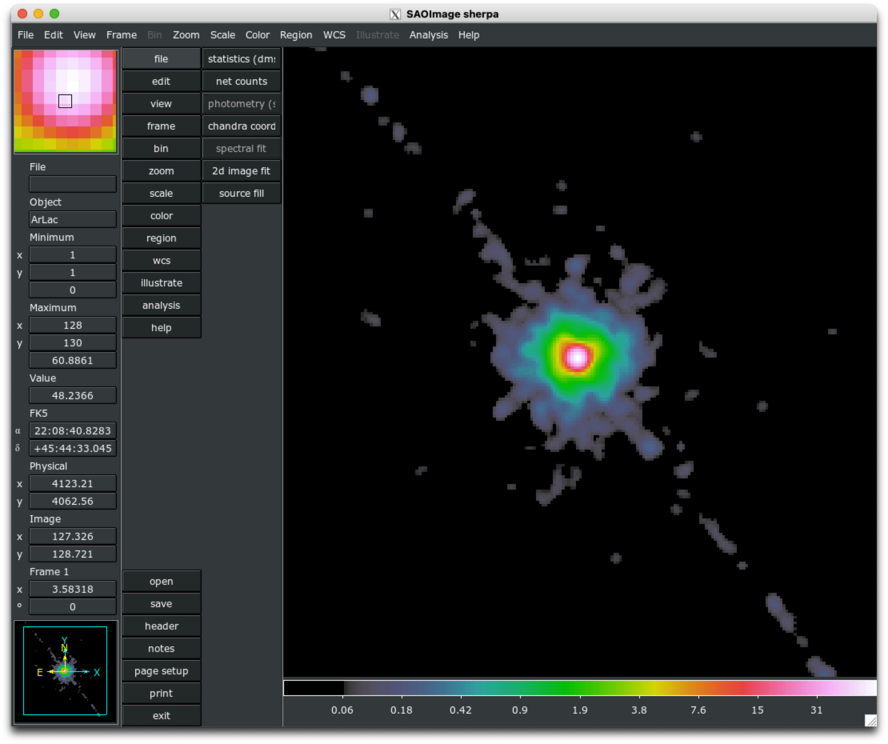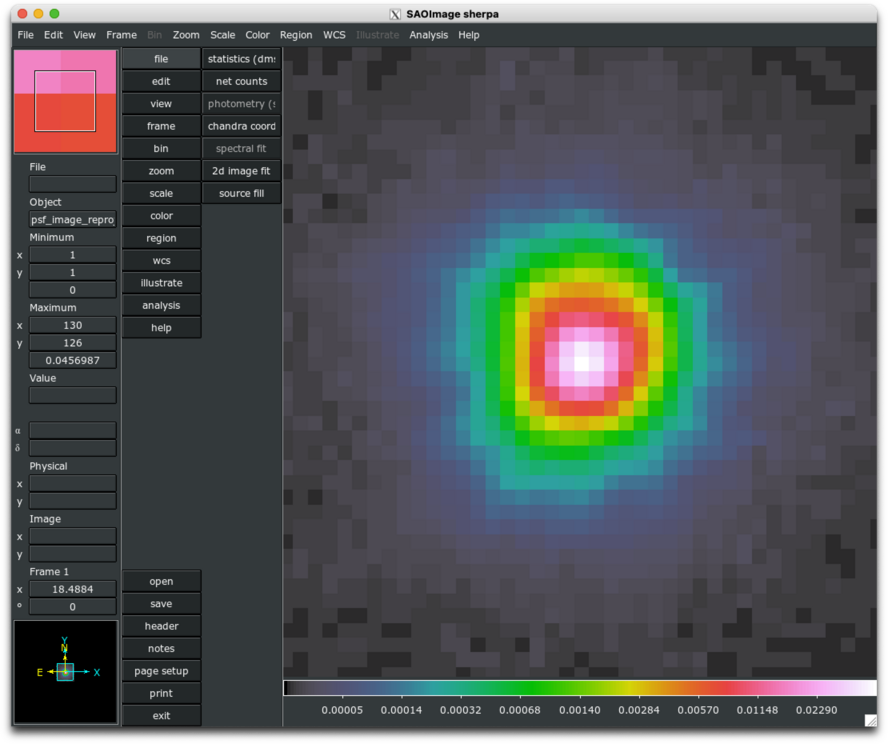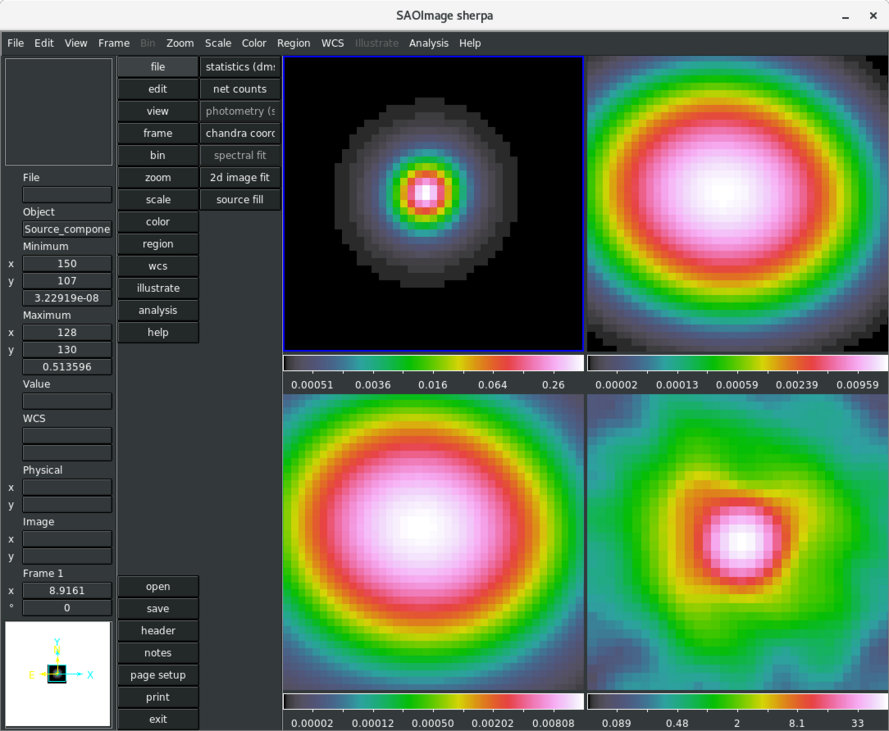# Fitting Image Data with Convolved and Unconvolved Model Components## Overview

#### Synopsis:

This thread demonstrates the use of the set_full_model functionality for manually defining a complete source model expression for fitting spatial 2-D data, where one or more components are convolved by a PSF model, and others are not. An exposure map file is input to Sherpa as a file-based exposure map model via the load_table_model function, and used in the fitting of all model components, both unconvolved extended emission and convolved point-source emission. A Point-Spread-Function (PSF) image is loaded via load_psf to be used as the convolution kernel for the point-source emission, only.

Last Update: 15 Feb 2023 - updated for CIAO 4.15, example revised using reproducible data sets.

## Reading and Plotting 2D FITS Data

In this thread we use 2D spatial data from the FITS data file src_image.fits, which was created by binning a Chandra events table in sky coordinates and filtering it to contain only the source region of interest—a square region of extended source emission containing a bright central point source. This data set is input to Sherpa with the load_image command, and can be visualized with the image_data or contour_data commands.

sherpa> load_image("src_image.fits")

sherpa> image_data()


The image_data command opens the source data image in DS9, as shown in Figure 1. In this example, each image pixel subtends 0.123 arcsec (sky coordinates binned by a factor of 0.25) for an ACIS observations of AR Lac (ObsID 9689).

unix% dmcopy infile="acisf09689_repro_evt2.fits[bin x=4091.5:4155.5:0.25,y=4030.5:4094.5:0.25]" src_image.fits


### Figure 1: Source data image### Figure 1: Source data image

Image of source data sent to DS9 by the Sherpa image_data function. The image has been smoothed in DS9 using a 2σ Gaussian.

The show_data command lists information available for this data set, such as the binning of the image, physical dimensions, and coordinate system.

sherpa> show_data()
Data Set: 1
Filter:
name      = src_image.fits
x0        = Float64
x1        = Float64
y         = Float64
shape     = (256, 256)
staterror = None
syserror  = None
sky       = physical
crval    = [4091.5,4030.5]
crpix    = [0.5,0.5]
cdelt    = [0.25,0.25]
eqpos     = world
crval    = [332.1753, 45.7471]
crpix    = [4096.5,4096.5]
cdelt    = [-0.0001, 0.0001]
crota    = 0
epoch    = 2000
equinox  = 2000
coord     = logical


## Defining a Source Model

In order to define a multi-component source model expression in Sherpa containing both convolved and unconvolved components with which to fit a single data set, the model must be manually defined using the set_full_model function (as opposed to the set_source function, which automatically convolves an entire source model expression with a PSF or response model). After examining Figure 1, a 2D β-model may be appropriate for describing the extended wings of the source emission, while a PSF-convolved 2D Gaussian model can be used to describe the central point-source component. We choose to apply an (optional) exposure map model to the full source model expression to account for a variable response across the data image, and convolve only the point-source model component by a PSF model. First, we set up the exposure map and PSF model components, and then define the full source model expression with set_full_model.

### Setting the exposure map

We define a file-based exposure map model by loading an exposure map file with the load_table_model command:

sherpa> load_table_model("emap", "9689_area_bin0.25_broad_thresh.expmap")

sherpa> freeze(emap)


To display the status of the model emap, use the print() command; notice that Sherpa identifies the exposure map model as "tablemodel.emap":

sherpa> print(emap)
tablemodel.emap
Param        Type          Value          Min          Max      Units
-----        ----          -----          ---          ---      -----
emap.ampl    frozen            1 -3.40282e+38  3.40282e+38


In this particular example, the exposure map has units of $$\mathrm{cm^{2} \cdot \frac{count}{photon}}$$ which may be generated using ' units=area' with fluximage or 'normalize=yes' with mkexpmap. The exposure map amplitude is frozen, since it's a fixed component in this model that characterizes the variation across the detector.

### Setting the PSF convolution model

A PSF instrument model may be established by loading an image of a PSF into Sherpa, e.g. one created with the Chandra Ray Tracer (ChaRT), or by defining a Sherpa model expression which describes the shape of the PSF. Both types of PSF models are added to the current list of models in the session using the load_psf command. In this example, we load an image containing a simulated on-axis Chandra PSF of AR Lac on [an ideal] HRC-I (as observed with the calibration observation ObsID 13182), reprojected to the same tangent-plane/sky coordinates defined in src_image.fits (using reproject_image).

sherpa> load_psf("psf0", "psf_image.fits")

sherpa> print(psf0)
psfmodel.psf0
Param        Type          Value          Min          Max      Units
-----        ----          -----          ---          ---      -----
psf0.kernel  frozen psf_image.fits
psf0.norm    frozen            1            0            1


Normally—i.e., when set_source is used to define a source model expression—the model to be used as the PSF convolution model must be defined as such with the set_psf command; however, it is not necessary in this case since we are manually defining the full source model expression using the set_full_model functionality, as shown in the next section of this thread. To learn how to fully establish a PSF model when defining a source model with set_source, see the Sherpa thread Accounting for PSF Effects in 2D Image Fitting.

While it is not strictly necessary to run the set_psf command in this context, it must be run in order to utilize most of the PSF-specific Sherpa commands, e.g. the image_psf command for viewing the PSF image in DS9. Below, we go ahead and run set_psf in order to visualize the PSF model:

sherpa> set_psf(psf0)

sherpa> image_psf()


The PSF image is shown in Figure 2.

### Figure 2: PSF image### Figure 2: PSF image

Running the set_psf command also allows us to take advantage of the show_psf and show_kernel commands, which offer more information than "print(psf0)":

sherpa> show_kernel()
PSF Kernel: 1
psfmodel.psf0
Param        Type          Value          Min          Max      Units
-----        ----          -----          ---          ---      -----
psf0.kernel  frozen psf_image_reproj.fits
psf0.size    frozen   (256, 256)   (256, 256)   (256, 256)
psf0.center  frozen   (128, 128)   (128, 128)   (128, 128)
psf0.norm    frozen            1            0            1

sherpa> show_psf()
PSF Model: 1
name      = psf_image_reproj.fits
x0        = Float64
x1        = Float64
y         = Float64
shape     = (256, 256)
staterror = None
syserror  = None
sky       = physical
crval    = [4091.5,4030.5]
crpix    = [0.5,0.5]
cdelt    = [0.25,0.25]
eqpos     = world
crval    = [332.1753, 45.7471]
crpix    = [4096.5,4096.5]
cdelt    = [-0.0001, 0.0001]
crota    = 0
epoch    = 2000
equinox  = 2000
coord     = logical


### Defining a manual source model with set_full_model

We define the complete source model expression using set_full_model as follows, where we also include a Sherpa const2d model component to describe the constant background level contributing to the total emission:

sherpa> set_coord("physical")

sherpa> set_full_model(emap*(const2d.bgnd + beta2d.ext + psf0(gauss2d.ps)))


Since the model parameters will be expressed in terms of 'physical' sky coordinates, the analysis coordinates is also set with set_coord.

In a set_full_model expression, any response or PSF convolution models which should be applied to one or more individual model components must be explicitly defined—in this example, the PSF convolution model named "psf0" is applied only to the 2D Gaussian model component used to describe the point-source emission by enclosing the gauss2d component ps with parentheses, e.g. psf0(gauss2d.ps) [or psf0(ps) if the ps model object has already been established].

We can print the default model parameter values for the defined model using show_model:

sherpa> show_model()
PSF Model: 1
name      = psf_image_reproj.fits
x0        = Float64
x1        = Float64
y         = Float64
shape     = (256, 256)
staterror = None
syserror  = None
sky       = physical
crval    = [4091.5,4030.5]
crpix    = [0.5,0.5]
cdelt    = [0.25,0.25]
eqpos     = world
crval    = [332.1753, 45.7471]
crpix    = [4096.5,4096.5]
cdelt    = [-0.0001, 0.0001]
crota    = 0
epoch    = 2000
equinox  = 2000
coord     = logical

Model: 1
(tablemodel.emap * ((const2d.bgnd + beta2d.ext) + psfmodel.psf0(gauss2d.ps)))
Param        Type          Value          Min          Max      Units
-----        ----          -----          ---          ---      -----
emap.ampl    thawed            1 -3.40282e+38  3.40282e+38
bgnd.c0      thawed            1 -3.40282e+38  3.40282e+38
ext.r0       thawed           10  1.17549e-38  3.40282e+38
ext.xpos     thawed            0 -3.40282e+38  3.40282e+38
ext.ypos     thawed            0 -3.40282e+38  3.40282e+38
ext.ellip    frozen            0            0        0.999
ext.theta    frozen            0     -6.28319      6.28319    radians
ext.ampl     thawed            1 -3.40282e+38  3.40282e+38
ext.alpha    thawed            1          -10           10
ps.fwhm      thawed           10  1.17549e-38  3.40282e+38
ps.xpos      thawed            0 -3.40282e+38  3.40282e+38
ps.ypos      thawed            0 -3.40282e+38  3.40282e+38
ps.ellip     frozen            0            0        0.999
ps.theta     frozen            0     -6.28319      6.28319    radians
ps.ampl      thawed            1 -3.40282e+38  3.40282e+38


Before fitting the model to the source, we can set some initial model parameter values:

sherpa> ext.xpos = 4123
sherpa> ext.ypos = 4062

sherpa> bgnd.c0 = 0.0004

sherpa> ps.theta.min = 0
sherpa> ps.theta.max = 2*np.pi

sherpa> ext.theta.min = 0
sherpa> ext.theta.max = 2*np.pi

sherpa> ext.ampl.min = 0
sherpa> bgnd.c0.min = 0

sherpa> freeze(bgnd)
sherpa> thaw(ps)
sherpa> thaw(ext)


Examining the PSF image, it can also be seen that the PSF center is not quite at the center of the image array. We can re-define the location of the PSF kernel in the image by setting the center attribute in image coordinates.

sherpa> psf0.center = [130, 125]


The updated model parameters can be viewed again with show_model:

sherpa> show_model()

{...psf model information shown above...}

Model: 1
(tablemodel.emap * ((const2d.bgnd + beta2d.ext) + psfmodel.psf0(gauss2d.ps)))
Param        Type          Value          Min          Max      Units
-----        ----          -----          ---          ---      -----
emap.ampl    frozen            1 -3.40282e+38  3.40282e+38
bgnd.c0      frozen       0.0004            0  3.40282e+38
ext.r0       thawed           10  1.17549e-38  3.40282e+38
ext.xpos     thawed         4123 -3.40282e+38  3.40282e+38
ext.ypos     thawed         4062 -3.40282e+38  3.40282e+38
ext.ellip    thawed            0            0        0.999
ext.theta    thawed            0            0      6.28319    radians
ext.ampl     thawed            1            0  3.40282e+38
ext.alpha    thawed            1          -10           10
ps.fwhm      thawed           10  1.17549e-38  3.40282e+38
ps.ampl      thawed            1            0  3.40282e+38


## Fitting the model

We can now fit the manually defined model to the data in the usual way using the fit command. In this example, we decide to fit with the Sherpa C-Stat statistic and the Nelder-Mead optimization method, which we specify using the set_stat and set_method commands:

sherpa> show_stat()
Statistic: Chi2Gehrels
Chi Squared with Gehrels variance.

The variance is estimated from the number of counts in each bin,
but unlike Chi2DataVar, the Gaussian approximation is not
used. This makes it more-suitable for use with low-count data.

The standard deviation for each bin is calculated using the
approximation from _:

sigma(i,S) = 1 + sqrt(N(i,s) + 0.75)

where the higher-order terms have been dropped. This is accurate
to approximately one percent. For data where the background has
not been subtracted then the error term is:

sigma(i) = sigma(i,S)

whereas with background subtraction,

sigma(i)^2 = sigma(i,S)^2 + [A(S)/A(B)]^2 sigma(i,B)^2

A(B) is the off-source "area", which could be
the size of the region from which the background is extracted, or
the length of a background time segment, or a product of the two,
etc.; and A(S) is the on-source "area". These terms may be defined
for a particular type of data: for example, PHA data sets A(B) to
BACKSCAL * EXPOSURE from the background data set and A(S) to
BACKSCAL * EXPOSURE from the source data set.

--------
Chi2DataVar, Chi2ModVar, Chi2XspecVar

Notes
-----
The accuracy of the error term when the background has been
subtracted has not been determined. A preferable approach to
background subtraction is to model the background as well as the
source signal.

References
----------

..  "Confidence limits for small numbers of events in
astrophysical data", Gehrels, N. 1986, ApJ, vol 303,
p. 336-346.

sherpa> show_method()
Optimization Method: LevMar
name    = levmar
ftol    = 1.19209289551e-07
xtol    = 1.19209289551e-07
gtol    = 1.19209289551e-07
maxfev  = None
epsfcn  = 1.19209289551e-07
factor  = 100.0
verbose = 0

sherpa> set_stat("cstat")


Before running the fit, we also further restrict the portion of the source image to explore parameter-space over with the notice2d using the chosen analysis coordinates.

sherpa> notice2d("box(4123.15,4062.65,11.5,11.5)")
dataset 1: Field() -> Box(4123.15,4062.65,11.5,11.5)

sherpa> fit()
Dataset               = 1
Statistic             = cstat
Initial fit statistic = 2.0825e+06
Final fit statistic   = 1983.46 at function evaluation 3902
Data points           = 2116
Degrees of freedom    = 2107
Probability [Q-value] = 0.97328
Reduced statistic     = 0.941365
Change in statistic   = 2.08051e+06
ext.r0         0.594816
ext.xpos       4123.36
ext.ypos       4062.99
ext.ellip      0.0802603
ext.theta      6.28143
ext.ampl       0.583803
ext.alpha      2.6106
ps.fwhm        3.85448
ps.ampl        0.0162965


The fit will be further refined by unlinking the Gaussian model components from the corresponding β-model components and thawing the background component. Note that unlinked model parameters return to their default values. In this example, the unlinked parameters' value are explicitly set to match the previously linked to parameters' fitted values.

sherpa> unlink(ps.xpos)
sherpa> ps.xpos = ext.xpos.val
sherpa> ps.ypos = ext.ypos.val

sherpa> ps.theta = ext.theta.val
sherpa> ps.ellip = ext.ellip.val

sherpa> thaw(bgnd)
sherpa> fit()

Dataset               = 1
Statistic             = cstat
Initial fit statistic = 1983.46
Final fit statistic   = 1949.15 at function evaluation 5358
Data points           = 2116
Degrees of freedom    = 2102
Probability [Q-value] = 0.991954
Reduced statistic     = 0.927282
Change in statistic   = 34.3098
bgnd.c0        0.00037978
ext.r0         0.694364
ext.xpos       4123.37
ext.ypos       4062.99
ext.ellip      0.040359
ext.theta      3.91472
ext.ampl       0.562667
ext.alpha      3.34236
ps.fwhm        3.91617
ps.xpos        4123.14
ps.ypos        4062.99
ps.ellip       0.179069
ps.theta       6.1531
ps.ampl        0.0192026


The image_model, image_fit, and image_resid commands can be used to display the complete model [expression] and fit residuals images from the set_full_model functionality and we can use the image_source_component command to separately plot each of the unconvolved model components contributing to the fit of the source emission:

sherpa> image_source_component(ext)
sherpa> image_source_component(ps, tile=True, newframe=True)
sherpa> image_source_component(psf0(ps), tile=True, newframe=True)
sherpa> image_data(tile=True, newframe=True)


These commands create the images displayed in Figure 3.

### Figure 3: Images of individual, fitted model components### Figure 3: Images of individual, fitted model components

DS9 display of images of the individual, fitted model components contributing to the full model description of the extended and point-source emission (some images have different scale and zoom settings for visual enhancement). In the upper-left corner of the display is the 2D β-model component fitted to the extended source emission; in the upper-right is the unconvolved 2D Gaussian component used to describe the central point source emission; in the lower-left is the PSF-convolved 2D Gaussian component; and in the lower-right is the smoothed data image for comparison.

It should be noted that for the series of commands issued above for creating the display in Figure 3 that normally the image_model_component command is used to plot convolved model components, where the PSF or response model associated with a given model component is automatically applied. Since we have defined our source model manually with set_full_model, we must also ensure that we manually convolve source model components within the image_source_model expression, as shown above for the PSF-convolved point-source component of the model.

## Saving a Sherpa Session

To save this Sherpa session in order to return to the analysis at a later point, the following commands may be used:

sherpa> save("manual_fit.save")

sherpa> save_all("manual_fit.ascii")


The save function records all the information about the current session to the binary file manual_fit.save, and the save_all function records the session settings to an editable ASCII file.

To restore the session that was saved to the binary file manual_fit.save or ASCII file manual_fit.ascii:

sherpa> restore("manual_fit.save")

sherpa> %run -i manual_fit.ascii


## Scripting It

The file fit.py is a Python script which performs the primary commands used above; it can be executed by typing %run -i fit.py on the Sherpa command line.

The Sherpa script command may be used to save everything typed on the command line in a Sherpa session:

sherpa> script(filename="sherpa.log", clobber=False)


(Note that restoring a Sherpa session from such a file could be problematic since it may include syntax errors, unwanted fitting trials, etcetera.)

## Summary

This thread is complete, so we can exit the Sherpa session:

sherpa> quit


## History

 10 Jan 2010 original version 06 Jan 2012 reviewed for CIAO 4.4 (no changes) 13 Dec 2012 reviewed for CIAO 4.5 (no changes) 06 Dec 2013 reviewed for CIAO 4.6: typos fixed 07 Apr 2015 reviewed for CIAO 4.7, no content change. 10 Dec 2015 reviewed for CIAO 4.8, no content change. 15 Feb 2023 updated for CIAO 4.15, example revised using reproducible data sets.